# Algebra

•  One Step Equation Game Have fun solving one-step equations by playing this interesting math basketball game.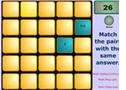Equation Game In this interactive concentration game, students will try to match each equation with the correct solution as fast as they can.Absolute Value Equations Do you know how to solve absolute value equations? Play this fun millionaire-style game to find out.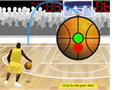Two-Step Equation Game Show off your equation solving skills by playing this interesting math basketball game. You can play it alone or in teams. Solve one step equations with Kiwi.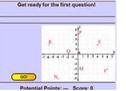Coordinate Plane Game Earn as many points as possible by quickly locating points in the coordinate plane.Systems of Equations GameSolve different systems of equations and have fun in the same time when playing this interactive game.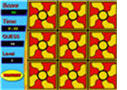Square Root Game In this interactive concentration game, students will match the square roots of perfect squares with the correct numbers.Slope-Intercept Game Do you know how to write equations in slope-intercept form? Play this fun game to check your knowledge.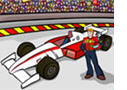Adding Integers Game Practice adding signed numbers by playing this fast-paced math racing game.Adding Integers Game Identify whether different sums are negative, zero, or positive by dragging problems in the correct basket.Subtracting Integers Game This is an interactive math racing game about subtracting signed numbers.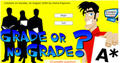Multiplying and Dividing Integers - Practice multiplying and dividing signed numbers.Operations with Integers Review the rules for adding, subtracting, multiplying, and dividing integers when playing this interactive jeopardy game.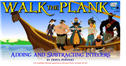Adding and Subtracting Integers - A fun way to practice adding and subtracting integers. Practice applying the six rules of exponents by playing this game with Kiwi.Square Roots Game This is a fun and interactive millionaire game about square roots.Rational and Irrational Numbers In this interesting game, middle school students will have fun classifying rational and irrational numbers.